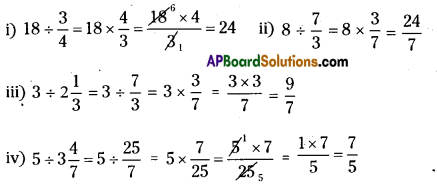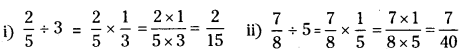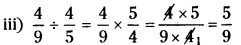AP State Syllabus AP Board 7th Class Maths Solutions Chapter 2 Fractions, Decimals and Rational Numbers Ex 4 Textbook Questions and Answers.

## AP State Syllabus 7th Class Maths Solutions 2nd Lesson Fractions, Decimals and Rational Numbers Exercise 4Question 1.
Find the reciprocal of each of the following fractions.
(i) $$\frac { 5 }{ 8 }$$
(ii) $$\frac { 8 }{ 7 }$$
(iii) $$\frac { 13 }{ 7 }$$
(iv) $$\frac { 3 }{ 4 }$$
Solution:
i) Reciprocal of $$\frac { 5 }{ 8 }$$ = $$\frac { 8 }{ 5 }$$
ii) Reciprocal of $$\frac { 8 }{ 7 }$$ = $$\frac { 7 }{ 8 }$$
iii) Reciprocal of $$\frac { 13 }{ 7 }$$ = $$\frac { 7 }{ 13 }$$
iv) Reciprocal of $$\frac { 3 }{ 4 }$$ = $$\frac { 4 }{ 3 }$$

Question 2.
Find
(i) 18 ÷ $$\frac { 3 }{ 4 }$$
(ii) 8 ÷ $$\frac { 7 }{ 3 }$$
(iii) 3 ÷ 2$$\frac { 1 }{ 3 }$$
(iv) 5 ÷ 3$$\frac { 4 }{ 7 }$$
Solution:Question 3.
Find
(i) $$\frac { 2 }{ 5 }$$ ÷ 3
(ii) $$\frac { 7 }{ 8 }$$ ÷ 5
(iii) $$\frac { 4 }{ 9 }$$ ÷ $$\frac { 4 }{ 5 }$$
Solution:Question 4.
Deepak can paint $$\frac { 2 }{ 5 }$$ of a house in one day. lfhe continues working at this rate, how many days will he take to paint the whole house?
Amount of work done by deepak in 1 day = $$\frac { 2 }{ 5 }$$ th
Total work can be done in = 1 ÷ $$\frac { 2 }{ 5 }$$ days
= $$\frac { 2 }{ 5 }$$ = $$1 \times \frac{5}{2}=\frac{1 \times 5}{2}=\frac{5}{2}=2 \frac{1}{2}$$ days.